Courses

# Statistical Physics NAT Level - 1

## 10 Questions MCQ Test Topic wise Tests for IIT JAM Physics | Statistical Physics NAT Level - 1

Description
This mock test of Statistical Physics NAT Level - 1 for IIT JAM helps you for every IIT JAM entrance exam. This contains 10 Multiple Choice Questions for IIT JAM Statistical Physics NAT Level - 1 (mcq) to study with solutions a complete question bank. The solved questions answers in this Statistical Physics NAT Level - 1 quiz give you a good mix of easy questions and tough questions. IIT JAM students definitely take this Statistical Physics NAT Level - 1 exercise for a better result in the exam. You can find other Statistical Physics NAT Level - 1 extra questions, long questions & short questions for IIT JAM on EduRev as well by searching above.
*Answer can only contain numeric values
QUESTION: 1

### A system of non-interacting Fermi particles with Fermi energy EF , has the density of states proportional to √E where E is the energy of a particles. The ratio of average energy per particle at T = 0 to the Fermi energy is.

Solution:

We know that E = U/N
and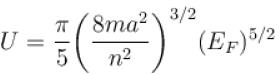and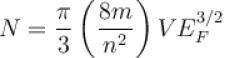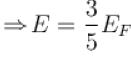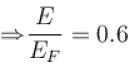*Answer can only contain numeric values
QUESTION: 2

### In a Maxwellian gas, if Vrms is the root means square velocity, then the most probable velocity  vmp in terms of vrms is.

Solution:

We know that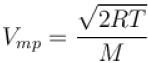and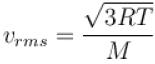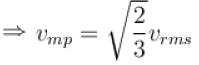vmp = 0.8165 vrms

*Answer can only contain numeric values
QUESTION: 3

### A system has energy level E0, 2E0, 3E0,....., where the excited states are triply degenerated. Three non-interacting bosons are placed in the system. The total energy of these bosons is 5E0, the number of microstates is.

Solution: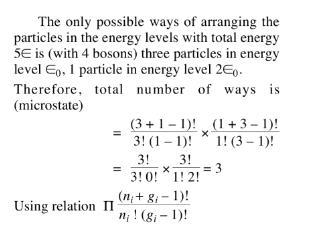*Answer can only contain numeric values
QUESTION: 4

The total number of accessible states of non-interacting particles of spin 1/2 is given that N = 20.

Solution:

Since the particles which exhibit odd integral spins are fermions and hence they obey Pauli’s exclusion principles. According to Pauli’s exclusion principle, not more than one particle can exist in the one quantum state. Hence, the number of assessible states N = 20.

*Answer can only contain numeric values
QUESTION: 5

The number of coordinates in the phase space of a single particle is.

Solution:

For a single particle, these are 3 degrees of position coordinates x, y, z and 3 degrees of momentum coordinates px, py, pz, hence total coordinates = 6.

*Answer can only contain numeric values
QUESTION: 6

The total energy per particle of a collection of free fermions is 3eV. The Fermi energy of the system (in eV) is?

Solution: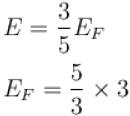= 5eV

*Answer can only contain numeric values
QUESTION: 7

An ensemble of N three level systems with energies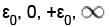in thermal equilibrium at temperature T. Let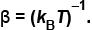If βε = 2, the probability of finding the system in level ε = 0 is given as  (1 + 2cosh 2)α . Find value of α?

Solution:

The probability of finding the system in the energy state with energy ε may be defined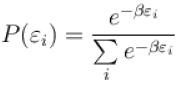But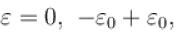hence probability of finding the sytem with ε = 0 is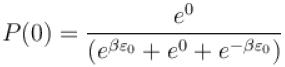In question, βε0 = 2 so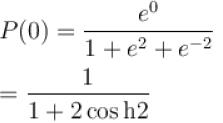P(0) = (1 + 2cosh 2)-1
⇒ α = 0.

*Answer can only contain numeric values
QUESTION: 8

The dimension of phase space of ten rigid diatomic molecule is

Solution:

For a rigid diatomic molecule, there is no rotation possible along the inter-nuclear axis. That remove one angle from 3 Euler angles needed to describe the orientation of the molecules, and hence also removes an angular momentum component. That leaves 4 angular degrees of freedom plus 3 coordinate and 3 momenta for the center of mass motions.
∴ One diatomic molecule has phase space of dimension 10, so the number ofphase space for10 diatomic molecule = 10 ×10 = 100.

*Answer can only contain numeric values
QUESTION: 9

For a particle moving in a circle of fixed radius with constant speed, dimension of phase space is.

Solution:

For a 2D moving in a plane as in case of a circular motion, we have two degrees of freedom for the q i.e. for x – y plane, q1 = x and q2 = y, and two degrees of freedom for the velocity given by ux and vy and thus px and py.
⇒ dimension of phase space = 2 + 2 = 4.

*Answer can only contain numeric values
QUESTION: 10

A system of N non-interacting classical point particles is constrained to move on the two-dimensional surface of a sphere. The internal energy of the system is in units of NkBT?

Solution:

Since the particle is moving on two dimensional surface, its degree of freedom = 2.
Hence, for N non-interacting particles total degree of freedom = 2N
The energy per degree of freedom = 1/2 kBT
Hence, total energy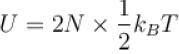U = NkBT